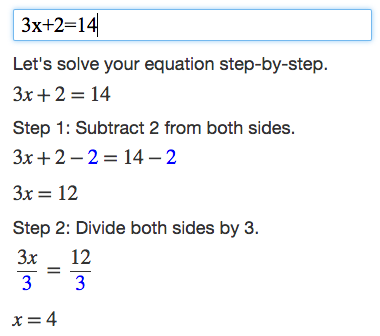Algebra Calculator

What do you want to calculate?

Examples: 1+2, 1/3+1/4, 2^3 * 2^2
(x+1)(x+2) (Simplify Example), 2x^2+2y @ x=5, y=3 (Evaluate Example)
y=x^2+1 (Graph Example), 4x+2=2(x+6) (Solve Example)

Algebra Calculator is a calculator that gives step-by-step help on algebra problems.

How to Use the Calculator

Type your algebra problem into the text box.

For example, enter 3x+2=14 into the text box to get a step-by-step explanation of how to solve 3x+2=14.More Examples

Trying the examples on the Examples page is the quickest way to learn how to use the calculator.

Math Symbols

If you would like to create your own math expressions, here are some symbols that the calculator understands:

- (Subtraction)
* (Multiplication)
/ (Division)
^ (Exponent: "raised to the power")
sqrt (Square Root) (Example: sqrt(9))

More Math Symbols

Tutorial

Read the full tutorial to learn how to graph equations and check your algebra homework.

Mobile App

Get the MathPapa mobile app! It works offline!